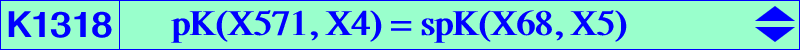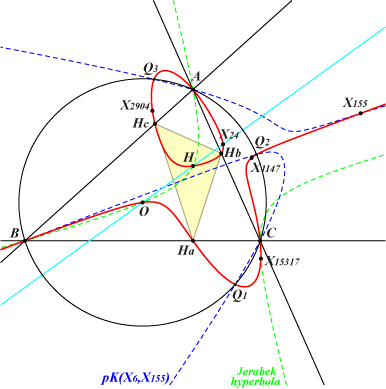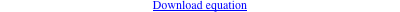too complicated to be written here. Click on the link to download a text file.X(3), X(4), X(24), X(155), X(1147), X(2904), X(15317), X(34756), X(53168), X(53169), X(53170), X(53171), X(53172) imaginary foci of the MacBeath inconic vertices of the orthic triangle infinite points of pK(X6,X68) points of pK(X6,X155) on (O) Geometric properties :K1318 is a MacBeath cubic as in Table 80. See also CL019. Its isogonal transform is K1337 = spK(X155, X5) = pK(X2165, X847). K1318 is the isogonal pK with pivot X(1147) with respect to the orthic triangle.